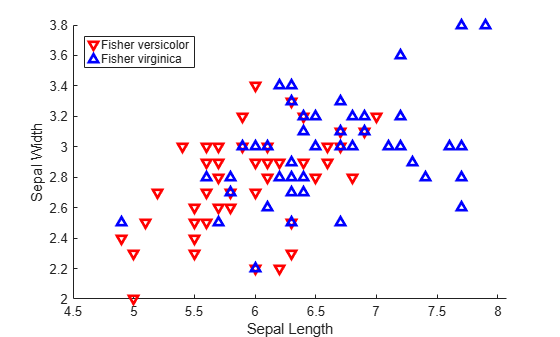Documentation

# classify

Discriminant analysis

## Syntax

class = classify(sample,training,group) class = classify(sample,training,group,'type') class = classify(sample,training,group,'type',prior) [class,err] = classify(...) [class,err,POSTERIOR] = classify(...) [class,err,POSTERIOR,logp] = classify(...) [class,err,POSTERIOR,logp,coeff] = classify(...) 

## Description

class = classify(sample,training,group) classifies each row of the data in sample into one of the groups in training. sample and training must be matrices with the same number of columns. group is a grouping variable for training. Its unique values define groups; each element defines the group to which the corresponding row of training belongs. group can be a categorical variable, a numeric vector, a character array, a string array, or a cell array of character vectors. training and group must have the same number of rows. classify treats <undefined> values, NaNs, empty character vectors, empty strings, and <missing> string values in group as missing data values, and ignores the corresponding rows of training. The output class indicates the group to which each row of sample has been assigned, and is of the same type as group.

class = classify(sample,training,group,'type') allows you to specify the type of discriminant function. Specify type inside single quotes. type is one of:

• linear — Fits a multivariate normal density to each group, with a pooled estimate of covariance. This is the default.

• diaglinear — Similar to linear, but with a diagonal covariance matrix estimate (naive Bayes classifiers).

• quadratic — Fits multivariate normal densities with covariance estimates stratified by group.

• diagquadratic — Similar to quadratic, but with a diagonal covariance matrix estimate (naive Bayes classifiers).

• mahalanobis — Uses Mahalanobis distances with stratified covariance estimates.

class = classify(sample,training,group,'type',prior) allows you to specify prior probabilities for the groups. prior is one of:

• A numeric vector the same length as the number of unique values in group (or the number of levels defined for group, if group is categorical). If group is numeric or categorical, the order of prior must correspond to the ordered values in group. Otherwise, the order of prior must correspond to the order of the first occurrence of the values in group.

• A 1-by-1 structure with fields:

• prob — A numeric vector.

• group — Of the same type as group, containing unique values indicating the groups to which the elements of prob correspond.

As a structure, prior can contain groups that do not appear in group. This can be useful if training is a subset a larger training set. classify ignores any groups that appear in the structure but not in the group array.

• The character vector or string scalar 'empirical', indicating that group prior probabilities should be estimated from the group relative frequencies in training.

prior defaults to a numeric vector of equal probabilities, i.e., a uniform distribution. prior is not used for discrimination by Mahalanobis distance, except for error rate calculation.

[class,err] = classify(...) also returns an estimate err of the misclassification error rate based on the training data. classify returns the apparent error rate, i.e., the percentage of observations in training that are misclassified, weighted by the prior probabilities for the groups.

[class,err,POSTERIOR] = classify(...) also returns a matrix POSTERIOR of estimates of the posterior probabilities that the jth training group was the source of the ith sample observation, i.e., Pr(group j|obs i). POSTERIOR is not computed for Mahalanobis discrimination.

[class,err,POSTERIOR,logp] = classify(...) also returns a vector logp containing estimates of the logarithms of the unconditional predictive probability density of the sample observations, p(obs i) = ∑p(obs i|group j)Pr(group j) over all groups. logp is not computed for Mahalanobis discrimination.

[class,err,POSTERIOR,logp,coeff] = classify(...) also returns a structure array coeff containing coefficients of the boundary curves between pairs of groups. Each element coeff(I,J) contains information for comparing group I to group J in the following fields:

• type — Type of discriminant function, from the type input.

• name1 — Name of the first group.

• name2 — Name of the second group.

• const — Constant term of the boundary equation (K)

• linear — Linear coefficients of the boundary equation (L)

• quadratic — Quadratic coefficient matrix of the boundary equation (Q)

For the linear and diaglinear types, the quadratic field is absent, and a row x from the sample array is classified into group I rather than group J if 0 < K+x*L. For the other types, x is classified into group I if 0 < K+x*L+x*Q*x'.

## Examples

collapse all

For training data, use Fisher's sepal measurements for iris versicolor and virginica:

load fisheriris SL = meas(51:end,1); SW = meas(51:end,2); group = species(51:end); h1 = gscatter(SL,SW,group,'rb','v^',[],'off'); set(h1,'LineWidth',2) legend('Fisher versicolor','Fisher virginica',... 'Location','NW')Classify a grid of measurements on the same scale:

[X,Y] = meshgrid(linspace(4.5,8),linspace(2,4)); X = X(:); Y = Y(:); [C,err,P,logp,coeff] = classify([X Y],[SL SW],... group,'Quadratic');

Visualize the classification:

hold on; gscatter(X,Y,C,'rb','.',1,'off'); K = coeff(1,2).const; L = coeff(1,2).linear; Q = coeff(1,2).quadratic; % Function to compute K + L*v + v'*Q*v for multiple vectors % v=[x;y]. Accepts x and y as scalars or column vectors. f = @(x,y) K + L(1)*x + L(2)*y + Q(1,1)*x.*x + (Q(1,2)+Q(2,1))*x.*y + Q(2,2)*y.*y; h2 = fimplicit(f,[4.5 8 2 4]); set(h2,'Color','m','LineWidth',2,'DisplayName','Decision Boundary') axis([4.5 8 2 4]) xlabel('Sepal Length') ylabel('Sepal Width') title('{\bf Classification with Fisher Training Data}')## Alternative Functionality

The fitcdiscr function also performs discriminant analysis. You can train a classifier by using the fitcdiscr function and predict labels of new data by using the predict function. The fitcdiscr supports cross-validation and hyperparameter optimization and does not require you to fit the classifier every time you make a new prediction or you change prior probabilities.

## References

 Krzanowski, W. J. Principles of Multivariate Analysis: A User's Perspective. New York: Oxford University Press, 1988.

 Seber, G. A. F. Multivariate Observations. Hoboken, NJ: John Wiley & Sons, Inc., 1984.